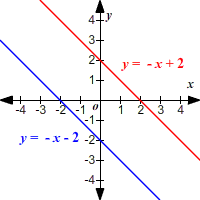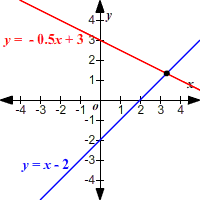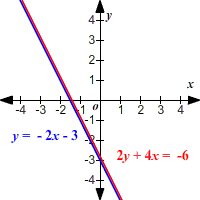# Solving Systems of Linear Equations Using Elimination

### Systems of Linear equations:

A system of linear equations is just a set of two or more linear equations.

In two variables $\left(x\text{\hspace{0.17em}}\text{\hspace{0.17em}}\text{and}\text{\hspace{0.17em}}\text{\hspace{0.17em}}y\right)$ , the graph of a system of two equations is a pair of lines in the plane.

There are three possibilities:

• The lines intersect at zero points. (The lines are parallel.)
• The lines intersect at exactly one point. (Most cases.)
• The lines intersect at infinitely many points. (The two equations represent the same line.)How to Solve a System of Linear Equations Using The Elimination Method (aka The Addition Method, aka The Linear Combination Method)

• Step $1$ : Add (or subtract) a multiple of one equation to (or from) the other equation, in such a way that either the $x$ -terms or the $y$ -terms cancel out.
• Step $2$ : Then solve for $x$ (or $y$ , whichever's left) and substitute back to get the other coordinate.

Now, how do we know that a linear equation obtained by the addition of the first equation with a scalar multiplication of the second is equivalent to the first?

Let us take an example. Consider the system

$\begin{array}{l}3x+2y=3\\ x-y=-4\end{array}$ .

Consider the equation obtained by multiplying the second equation by a constant $m$ and then adding the resultant equation with the first one.

That is, $\left(3x+2y\right)+m\left(x-y\right)=\left(3\right)+m\left(-4\right)$ .

What we need to prove is that this equation is equivalent to the equation $3x+2y=3$ .

We have $x-y=-4⇒m\left(x-y\right)=-4m$ .

Since $m\left(x-y\right)=-4m$ , subtract $m\left(x-y\right)$ from the left side and $-4m$ from the right side of the equation $\left(3x+2y\right)+m\left(x-y\right)=\left(3\right)-4m$ which will retain the balance.

$\left(3x+2y\right)+m\left(x-y\right)-m\left(x-y\right)=\left(3\right)-4m-\left(-4m\right)$

Cancelling common terms we get, $3x+2y=3$ which is equivalent to the first equation.

Therefore, the systems of equations $\begin{array}{l}3x+2y=3\\ x-y=-4\end{array}$ and $\begin{array}{l}\left(3x+2y\right)+m\left(x-y\right)=3+m\left(-4\right)\\ \text{\hspace{0.17em}}\text{\hspace{0.17em}}\text{\hspace{0.17em}}\text{\hspace{0.17em}}\text{\hspace{0.17em}}\text{\hspace{0.17em}}\text{\hspace{0.17em}}\text{\hspace{0.17em}}\text{\hspace{0.17em}}\text{\hspace{0.17em}}\text{\hspace{0.17em}}\text{\hspace{0.17em}}\text{\hspace{0.17em}}\text{\hspace{0.17em}}\text{\hspace{0.17em}}\text{\hspace{0.17em}}\text{\hspace{0.17em}}\text{\hspace{0.17em}}\text{\hspace{0.17em}}\text{\hspace{0.17em}}\text{\hspace{0.17em}}\text{\hspace{0.17em}}\text{\hspace{0.17em}}\text{\hspace{0.17em}}\text{\hspace{0.17em}}\text{\hspace{0.17em}}\text{\hspace{0.17em}}\text{\hspace{0.17em}}\text{\hspace{0.17em}}\text{\hspace{0.17em}}\text{\hspace{0.17em}}\text{\hspace{0.17em}}\text{\hspace{0.17em}}x-y=-4\end{array}$ are equivalent.

In general, for any system of equations $K=L$ and $P=Q$ , it ca be shown that $K+mP=L+mQ$ is equivalent to $K=L$ .

Example:

Solve the system $\left\{\begin{array}{l}4x+3y=-2\\ 8x-2y=12\end{array}$

Multiply the first equation by $-2$ and add the result to the second equation.

$\begin{array}{l}-8x-6y=4\\ \underset{_}{\text{\hspace{0.17em}}\text{\hspace{0.17em}}\text{\hspace{0.17em}}8x-2y=12}\\ \text{\hspace{0.17em}}\text{\hspace{0.17em}}\text{\hspace{0.17em}}\text{\hspace{0.17em}}\text{\hspace{0.17em}}\text{\hspace{0.17em}}\text{\hspace{0.17em}}\text{\hspace{0.17em}}\text{\hspace{0.17em}}-8y=16\end{array}$

Solve for $y$ .

$y=-2$

Substitute for $y$ in either of the original equations and solve for $x$ .

$\begin{array}{l}4x+3\left(-2\right)=-2\\ 4x-6=-2\\ 4x=4\\ x=1\end{array}$

The solution is $\left(1,-2\right)$ .